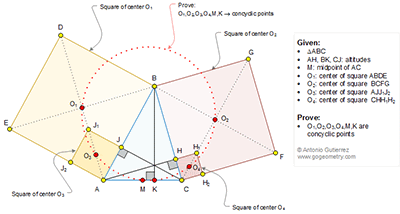Friday, December 9, 2016

Geometry Problem 1294 Triangle, Altitudes, Four Squares, Center, Midpoint, Concyclic Points

Geometry Problem. Post your solution in the comment box below.
Level: Mathematics Education, High School, Honors Geometry, College.

Details: Click on the figure below.1.Problem 1294
Is <AO_1B+<AKB=90+90=180,then A,K,B and O_1 are concyclic.So <O_1KA=O_1BA=45,but AO_3=O_3J_1, AM=MC so MO_3//CJ_1 ,(CJ is perpendicular in AB ) then MO_3 is perpendicular in AB.Is <MO_3A=<CJ_1A=45=<O_1KA.Therefore the O_1,O_3,M and K are concyclic.Νow <CJB=90=<CO_2B then the point C,J,B and O_2 are concyclic.So
<CJO_2=<CBO_2=45,<AJC=90 and <O_3JA=45 then <O_3JA+<AJC+<CJO_2=180 so the
Point O_3,J and O_2 are collinear. Τherefore <O_2O_3O_1=90 . Similar <O_2O_4O_1=90.
So the point O_1,O_2, O_4 and O_3 are concyclic.Now <O_1KA=45=<O_2KC so <O_1KO_2=90=<O_1O_3O_2=O_1O_4O_2 then the point O_1,O_2, K,O_4 and O_2 are
Concyclic.Therefore the points O_1, O_2,O_3,O_4, M and K are concyclic.
APOSTOLIS MANOLOUDIS 4 HIGH SCHOOL KORYDALLOS PIRAEUS GREECE

2.AO1BK is concyclic so
< AKO1 = < ABO1 = 45

Now MO3 // CJ1 by applying the midpoint theorem to Tr. ACJ1.
Hence < AO3M = < AJ1C = 45

So < AKO1 = 45 = < AO3M.
So O1O3MK is concyclic

Similarly we can show that O2O4KM is concyclic and so the result follows.

Sumith Peiris
Moratuwa
Sri Lanka

1.Sumith

In my opinion, concyclic of O1,O3,M,K and concyclic of O2,O4,K,M are not enough to conclude that O1,O2,O3,O4,K and M are concyclic. Please explain.
Peter

2.Peter